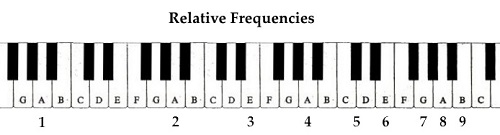# Log24

## Tuesday, September 23, 2014

### Simple Tune

Filed under: General — Tags: — m759 @ 7:00 PM

1  2  3  4  5  9  8  6  7220 * (1/4) =   55 = A1

220 * (2/4) = 110 = A2

220 * (3/4) = 165 = approximately E3 (164.8)

220 * (4/4) = 220 = A3

220 * (5/4) = 275 = approximately C4/D4 (277.2)

220 * (6/4) = 330 = approximately E4  (329.6)

220 * (7/4) = 385 = approximately G4  (392.0)

220 * (8/4) = 440 = A4

220 * (9/4) = 495 = approximately B4  (493.9)

Exact frequencies (such as 277.2) are from Wikipedia’s Piano key frequencies.

“It may be quite simple, but now that it’s done….